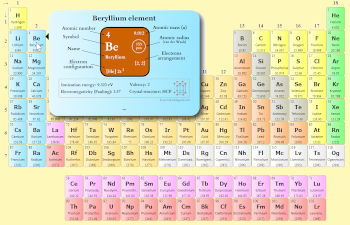# Periods in Periodic table Explained! (With 1-7 Period Names)

Periods in Periodic table: The periods are the horizontal rows on a Periodic table. There are 7 horizontal rows on Periodic table. Hence there are total 7 periods on the Periodic table.

So you have seen the names of periods in periodic table, Right?

Now let me give you very short and simple information about each Period with period names.

Let’s dive straight into it.

## Periods in Periodic Table (With Period Names)

There are total 7 periods in Periodic Table.

Let me explain each of these Periods in short.See how this Interactive Periodic Table helps you

• You can effortlessly find every single detail about the elements from this single Interactive Periodic table.
• You will get the detailed information about the periodic table which will convert a newbie into pro.
• You will also get the HD images of the Periodic table (for FREE).

Visit ➢ Periodic table

### Period 1: Shortest period

Period 1 of the periodic table is given the name shortest period because there are only two elements in period 1.

The elements of the shortest period are shown in table below.

Shortest period elements (Period 1)

Elements of period 1 possess only one energy shell.

### Period 2 and 3: Short period

Period 2 and period 3 of the periodic table are named as the short period because there are 8 elements in these periods.

The elements of the short period are shown in tables below.

Short period elements (Period 2)

All the elements of period 2 have two energy shells (or orbits).

Short period elements (Period 3)

All the elements of period 3 have three energy shells.

### Period 4 and 5: Long period

Period 4 and period 5 are given the name long periods of the periodic table because there are 18 elements in these periods.

The elements of the long periods are shown in tables below.

Long period elements (Period 4)

All the above elements of period 4 possess four energy shells.

Long period elements (Period 5)

These elements of period 5 have five shells in which the electrons revolve.

### Period 6 and 7: Longest period

Period 5 and period 6 are named as longest periods of the periodic table because there are 32 elements in these periods.

The elements of the longest periods are shown in tables below.

Longest period elements (Period 6)

All the above elements of period 6 possess six energy shells.

Longest period elements (Period 7)

These elements of the 7th period have seven energy shells for the electrons to revolve.

## Summary

As a summary of the whole topic, you should remember following points for the Periods of Periodic table.

• The horizontal rows on the periodic table are known as Periods.
• There are total 7 periods on the periodic table.
• The names of the periods in periodic table are;
• Period 1: Shortest period
• Period 2 and 3: Short period
• Period 4 and 5: Long period
• Period 6 and 7: Longest period
• The elements  in same Periods have the same number of energy shells (or energy orbits).

## Free Gift for you: Interactive Periodic Table

Let me tell you how this Interactive Periodic Table will help you in your studies.

1).
You can effortlessly find every single detail about the elements from this single Interactive Periodic table.

(For Interactive Periodic table, view on laptop/desktop for better experience. If you are on mobile device, then use a “Desktop site mode” to see interactive periodic table)

2). You will get the detailed information about the periodic table which will convert a newbie into pro.

3). You will also get the HD images of the Periodic table (for FREE).

Checkout Interactive Periodic table and download it’s high resolution image now (It’s FREE)